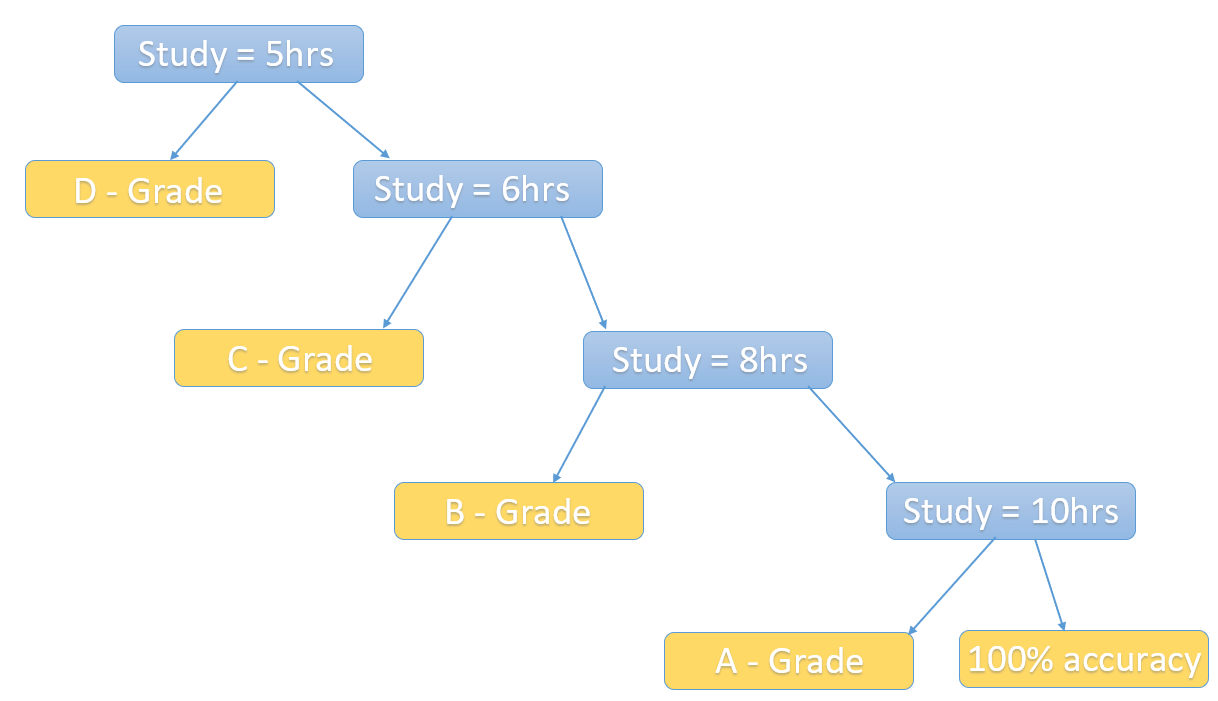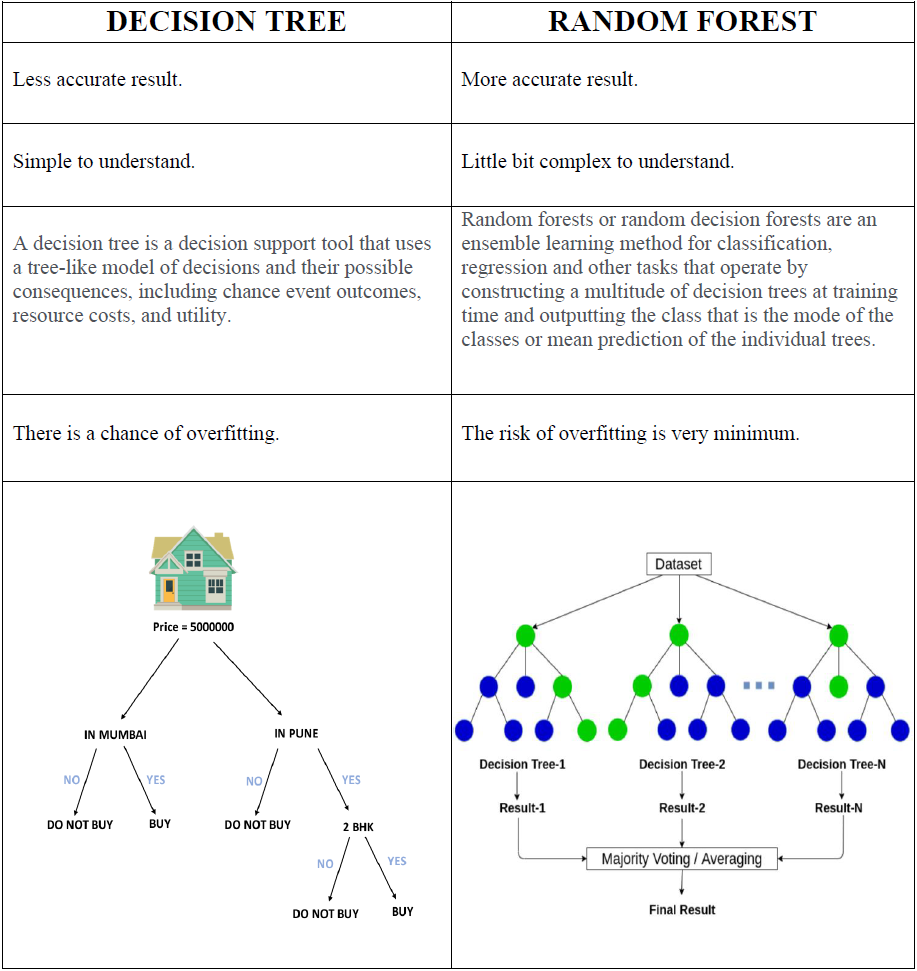## InfinityCodeX

Check out our blogs where we cover topics such as Python, Data Science, Machine Learning, Deep Learning. A Best place to start your AI career for beginner, intermediate peoples.

# Top 10 Strategies Which Will Make You King Of RANDOM FORESTFOREST

Random Forest is one of the Supervised Learning Techniques which is used to solve the Classification and Regression problem.

Evolution only comes when we found the limitations of something.

In this era of complete evolution, humans had found the limitations themselves. To overcome those limitations we try to create multiple solutions and go plus ultra.

To understand Random Forest completely, Let’s first understand what is Regression and Classification.

RegressionRegression is a method or an algorithm in Machine Learning that models a target value based on independent predictors. It is essentially a statistical tool used in finding out the relationship between a dependent variable an independent variable.

- Here we predict the output value as a specific number.

- In Regression, a Regression Tree is used when the target variable is numerical or continuous in nature.

- We fit a regression model to target variables using each of the independent variables.

- Each split is made based on the sum of the square error.REGRESSION

Classification: Classification is a process of categorizing a structured or a nonstructured set of data in different classes based on certain features/categories.CLASSIFICATION

The limitations of Decision Tree leads us to create a Random Forest. Let us see a quick overview of limitations of Decision Tree.

The Primary disadvantages of the decision tree are overfitting. Overfitting occurs when the algorithm capture noise in the data.

* In the Decision Tree there is also a problem of high variance. The model can get unstable due to small variance in the data.

* In a decision tree is there is Low Biased tree. A highly complicated decision tree tends to have a low bias which makes it difficult for the model to work with new data.

* Calculation can get very complex particularly if many values are uncertain and if many outcomes are linked.

To overcome all the limitations which we had in the Decision Tree. We will be using Random Forest. After all, this algorithm creates the forest with n number of Decision Trees.DECISION TREE AND RANDOM FOREST

In general more trees in the forest more robust the prediction and thus higher accuracy.

To understand this the concept clearly, here are some of the links which will help you to become not only King but KingKong of  Random Forest.KING KONG

So before diving into Random Forest directly, here is the map that will help you become King.

### (1) Ensemble Learning Overview

Ensemble Learning is used in multiple learning algorithms at the same time to obtain predictions with an aim to have better predictions than the individual model.

#### Q.) Why use Ensemble Learning?

* Gives us better accuracy which means the error will be minimum.

* It avoids overfitting so the consistency is very high.

* The bias and variance errors are also reduced to a minimum.

#### Q.) When and Where to use Ensemble Models?

*When a single model like Decision Tree over fits we can use Random Forest or we can use Ensemble of multiple similar models.

*It can be used for both Classification and Regression problems.

### (2) Why Random Forest?

It’s always important to understand, why we use any of the algorithms, for this article we will be talking about why we use Random Forest over the other algorithms.
Random Forest is an algorithm that helps us to get optimal output. It avoids overfitting, if you use multiple trees it automatically avoid the risk of overfitting.

For those who don’t know overfitting :

When a model performs well at training data but performs badly at test data.

In Random Forest the training time of the data is less.
The accuracy of the Random Forest is very high.
Random Forest also runs well especially in the larger database, for large data it produces highly accurate prediction in today's world of Big Data it is really very important. This is why Random Forest really comes in to help us.
It estimates the missing data, Data on today's world is very messy so when you have Random Forest for us which can maintain the accuracy when a large proportion of the data is missing.

### (3) What Is Random Forest?

Random Forest is a type of ensemble learning method. It is a versatile algorithm capable of performing both Regression and Classification.
Random Forest or Random Decision Tree Forest is the method that operates by constructing multiple Decision Trees during the training phase.

The decision of the majority of the trees is chosen by the Random Forest at the Final decision.
Random Forest is basically used for predictive modeling and machine learning technique.

### (4) Decision Tree VS Random ForestDECISION TREE VS RANDOM FOREST

### (5) Application Of Random Forest

There are multiple application of Random Fores but some of them are :

### (6) Features Of Random Forest

Here are the features of Random Forest

### (7) Disadvantages Of Random Forest

It’s not like that Random Forest is the perfect algorithm, everything has its disadvantages one way or other.

* More accurate ensemble require more trees, which means building and testing the model is a slower process.

* Random Forest is very good at Classification in comparison to Regression.

* In Regression Random Fores does not predict beyond the range of training data.

* Random Forest has been observed to overfit for some of the datasets with very noisy classification / regression tasks.

* For data including categorical variables with different number of levels, Random Forests are biased in favor of those attributes with more levels.

### (8) How Does Random Forest Algorithm Works?

In Random Forest we grow multiple trees as opposed to a single tree in the CART model. To classify new objects based on attribute each tree gives a classification and we say the tree votes for that class.
The forest choose the classification having the most votes over all the other trees in the forests and in the case of regression, it takes the average of the outputs by different trees.
Steps related to working of the Random Forest Algorithm :

T: Number of Trees to be constructed.
N: Number of Features.
O: The class with the highest vote.

#### (i) Select Random Features :

The first step is to actually, fill certain “m” features from N, where m less than N. As we know N is the total number of features, out of those features we will be selecting some random features out of those. The reason we are selecting certain features only is because if we select all the predictive variable that each of the Decision Tree will be the same and because of that our model is not learning something new.

#### (ii) Calculate Best Splitting Point :

For node d, calculate the best split point among them features. Here we pick up the most significant variable and then we split that particular node into further child nodes.

#### (iii) Split into n daughter nodes :

Split the node into n number of daughter nodes using the best splits.

#### (iv) Repeat the initial steps :

Repeat the first 3 steps until n number of nodes has been reached. Which means we have to repeat it until we rich the leaf nodes of the tree.

#### (v) Built your Forest :

Built your forest by repeating steps (i) till (iv) for T number of times.

After this (iv) steps we will have our 1 Decision Tree but Random Forest is about multiple Decision Trees.

This is not over yet, your final task will be to compile the results of all the Decision Trees and you will make a majority voting for the final result.

### (9) Random Forest Algorithm Example In Python

Using Random Forest we will predict the person will get a loan or not is not, in this first, we will use a Decision Tree and then a Random Forest, in the end, we will compare both of them in term of accuracy.

DATA SET: Loan_dataset

#Importing Important Libraries

import pandas as pd
import numpy as np
import seaborn as sns
import matplotlib.pyplot as plt
%matplotlib inline

#Importing Dataset

data = pd.read_csv(r"D:\Dig\lending_club_data01.csv")
data.head()
Output :

#Getting Information of the Dataset

data.info()
Output :

#Overall Description of the Dataset

data.describe()
Output :

#Referring bad_loans column we create good_loans column where we say yes if 0 and no if 1

data['good_loans']=data['bad_loans'].apply(lambda y: 'yes' if y==0 else 'no')
data.head()
Output :

#For training: all columns except bad_loans and good_loans
#For testing: good loans

X=data.drop(['bad_loans','good_loans'],1)
y=data['good_loans']
print(X.shape,y.shape)
Output :
`(1468, 7) (1468,)`

# Split data into training and testing

from sklearn.model_selection import train_test_split
X_train , X_test , y_train , y_test = train_test_split(X,y,test_size=0.2)
print(X_train.shape,X_test.shape,y_train.shape,y_test.shape)
Output :

(1174, 7) (294, 7) (1174,) (294,)

# Decision Tree :

from sklearn.tree import DecisionTreeClassifier
model=DecisionTreeClassifier()
model.fit(X_train,y_train)
Output :

#Evaluate our model

predict = model.predict(X_test)
from sklearn.metrics import classification_report, confusion_matrix
print(confusion_matrix(y_test,predict))
Output :
[[ 16 46]
` [ 38 194]]`

#Result
print(classification_report(y_test,predict))
Output :

Decision Tree Accuracy is 71%

# Random Forest :

from sklearn.ensemble import RandomForestClassifier
rf_model=RandomForestClassifier(n_estimators=250)
rf_model.fit(X_train,y_train)
Output :

print(X_train.shape,X_test.shape,y_train.shape,y_test.shape)
Output :

`(1174, 7) (294, 7) (1174,) (294,)`

#Evaluate our model

rf_predict = rf_model.predict(X_test)
print(confusion_matrix(y_test,rf_predict))
Output :

```[[ 10  52]
[  8 224]]```

#Result

print(classification_report(y_test,rf_predict))
Output :

Random Forest Accuracy is 80%

Hence it is proved that Random Forest is more accurate than the Decision Tree.

### * Random Forest Conclusion:CONCLUSION OF RANDOM FOREST

Did I Miss Anything?

Now I'd like to hear from you:

- Do you think Random Forest is awesome?

- What else would you like us to cover on this topic?

- What are the 5 most informative concepts you found in this blog?

Do comment your answers, and don't forget to share it with your friend so you can discuss more about this topic.
Top 10 Strategies Which Will Make You King Of RANDOM FORESTReviewed by InfinityCodeX on Thursday, June 04, 2020 Rating: 5

#### No comments:

No Spamming and No Offensive Language